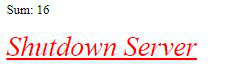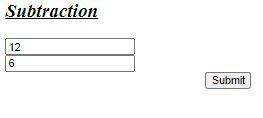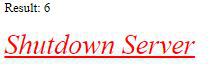Open In App

# Perform addition and subtraction using CherryPy

CherryPy also known as a web application library is a Python web framework that provides a friendly interface to the HTTP protocol for Python developers. It allows developers to build web applications the same way as in traditional object-oriented Python programs. Thereby, resulting in smaller source code developed in no time.

This framework is mainly for the developers who want to create a portable database-driven web application using Python, as it provides Create, Retrieve, Update and Delete functionalities.

The basic requirements for the installation of CherryPy include:

• Python with version 2.4 or above
• Cherrypy 3.0

### Installation

To install cherrypy run the following command in terminal:

```pip install cherrypy
```

Approach:

1. Create user interface to take input from user.
2. Write cherrypy program to perform required operations

HTML code to take input from the user-

## HTML

 `<``html``>``<``head``>``  ` `  ````<``body``>`` ` `  ``<``div` `class``=``"container"``>  ``    ``<``h2``><``u``><``i``>Operation``    ``<``form` `action``=``"store"` `id``=``"form"` `method``=``"GET"``>``    ``<``input` `type``=``"number"` `name``=``"number1"` `/><``br` `/>``    ``<``input` `type``=``"number"` `name``=``"number2"` `/><``br` `/>`` ` ` ` `    ``<``input` `style``=``"margin-left: 250px;"` `id``=``" submit"` `type``=``"submit"``/>``  ``    ``    ````  ````             ` ````

## Python3

 `import` `cherrypy`` ` ` ` `class` `Root(``object``):``    ` `    ``@cherrypy``.expose``    ``def` `index(``self``):``        ``return` `"""````  ` `  `````` ` `  ``
``    ``

``    ``
``    ``
``    ``
`` ` ` ` `    ``
``  ``    ``    ````  ````             ` ```"""``    ` `     ` `    ``@cherrypy``.expose``    ``def` `store(``self``, number1, number2):``        ``num1``=``int``(number1)``        ``num2``=``int``(number2)``        ``answer``=``num1``+``num2``       ` `        ``out``=` `"""``        ````         ` `         ` `

Sum: %s

`` ` `        ` `         ` `        ``Shutdown Server``        ````        ````         ` `        ``"""``        ` `        ``return` `out ``%` `(``str``(answer))``   ` `     ` `    ``@cherrypy``.expose``    ``def` `shutdown(``self``):``        ``cherrypy.engine.exit()`` ` `if` `__name__``=``=``"__main__"``:``    ``cherrypy.config.update({``'server.socket_port'``: ``8087``})``     ` `    ``cherrypy.quickstart(Root())`

Output:Code for subtraction

## Python3

 `import` `cherrypy`` ` ` ` `class` `Root(``object``):``    ` `    ``@cherrypy``.expose``    ``def` `index(``self``):``        ``return` `"""````  ` `  `````` ` `  ``
``    ``

Subtraction

``    ``
``    ``
``    ``
`` ` ` ` `    ``
``  ``    ``    ````  ````             ` ```"""``    ` `     ` `    ``@cherrypy``.expose``    ``def` `store(``self``, number1, number2):``        ``num1``=``int``(number1)``        ``num2``=``int``(number2)``        ``answer``=``num1``-``num2``       ` `        ``out``=` `"""``        ````         ` `         ` `

Result: %s

`` ` `        ` `         ` `        ``Shutdown Server``        ````        ````         ` `        ``"""``        ` `        ``return` `out ``%` `(``str``(answer))``   ` `     ` `    ``@cherrypy``.expose``    ``def` `shutdown(``self``):``        ``cherrypy.engine.exit()`` ` `if` `__name__``=``=``"__main__"``:``    ``cherrypy.config.update({``'server.socket_port'``: ``8087``})``     ` `    ``cherrypy.quickstart(Root())`

Output: